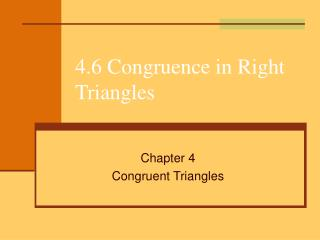DownloadDownload Presentation4.6 Congruence in Right Triangles

# 4.6 Congruence in Right Triangles

Télécharger la présentation## 4.6 Congruence in Right Triangles

- - - - - - - - - - - - - - - - - - - - - - - - - - - E N D - - - - - - - - - - - - - - - - - - - - - - - - - - -
##### Presentation Transcript

1. 4.6 Congruence in Right Triangles Chapter 4 Congruent Triangles

2. 4.6 Congruence in Right Triangles Right Triangle Hypotenuse Leg Leg *The Hypotenuse is the longest side and is always across from the right angle*

3. Pythagorean Theorem a2 + b2 = c2 c *c is always the hypotenuse a b

4. Pythagorean Theorem a2 + b2 = c2 c *c is always the hypotenuse 3 4

5. Pythagorean Theorem a2 + b2 = c2 13 *c is always the hypotenuse a 5

6. Pythagorean Theorem 25 25 7 7 Are these triangles congruent?

7. Congruence in Right Triangles Theorem 4-6 Hypotenuse-Leg (H-L) Theorem If the hypotenuse and a leg of one right triangle are congruent to the hypotenuse and a leg of another right triangle, then the triangles are congruent.

8. Congruence in Right Triangles Are the two triangles congruent? A X B C Y Z

9. Proving Triangles Congruent Given: WJ = KZ, <W and <K are right angles Prove:ΔJWZ = ΔZKJ Z W J K

10. Proving Triangles Congruent Given: CD = EA, AD is the perpendicular bisector of CE Prove: ΔCBD = ΔEBA C D A B E

11. Practice • Pg 219 1-4 Write a two-column proof • Pg 219 5-8 Answer Question • Pg 220 9 - 10 Answer Question • Pg 220 11-12 Write a two-column proof • Pg 220-221 14-17 • Pg 222 28 – 29 Write a two-column proof